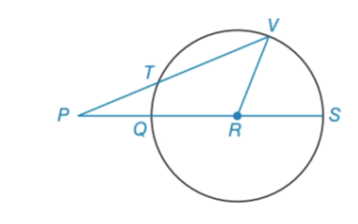Chapter 6.2, Problem 38E### Elementary Geometry for College St...

6th Edition
Daniel C. Alexander + 1 other
ISBN: 9781285195698

#### Solutions

Chapter
Section### Elementary Geometry for College St...

6th Edition
Daniel C. Alexander + 1 other
ISBN: 9781285195698
Textbook Problem
1 views

# In ⊙ R ,   Q S = 2 ( P T ) . Also, m ∠ P = 23 ° . Find m ∠ V R S .To determine

To find:

mVRS.

Explanation

Given:

Calculation:

Consider the given figure and Draw RT¯.

Given that,

QS=2(PT)

mP=23°

ΔPTR is isosceles.

PT=RT

Therefore,

mTRP=23°mTQ=mTRP=23°

P has the vertex outside the circle

### Still sussing out bartleby?

Check out a sample textbook solution.

See a sample solution

#### The Solution to Your Study Problems

Bartleby provides explanations to thousands of textbook problems written by our experts, many with advanced degrees!

Get Started

#### In Exercises 23-36, find the domain of the function. 26. g(x)=2x+1x1

Applied Calculus for the Managerial, Life, and Social Sciences: A Brief Approach

#### A sample with a mean of M = 8 has X = 56. How many scores are in the sample?

Essentials of Statistics for The Behavioral Sciences (MindTap Course List)

#### In problems 5-8, write the following sets in a second way. 7.

Mathematical Applications for the Management, Life, and Social Sciences

#### Subtract 1sinsin.

Trigonometry (MindTap Course List)

#### True or False:

Study Guide for Stewart's Single Variable Calculus: Early Transcendentals, 8th

#### Express 5.037 as a percent.

Mathematics For Machine Technology